1. /
2. CBSE
3. /
4. Class 07
5. /
6. Mathematics
7. /
8. NCERT Solutions for Class...

# NCERT Solutions for Class 7 Maths Exercise 10.6### myCBSEguide App

Download the app to get CBSE Sample Papers 2023-24, NCERT Solutions (Revised), Most Important Questions, Previous Year Question Bank, Mock Tests, and Detailed Notes.

NCERT solutions for Maths Practical Geometry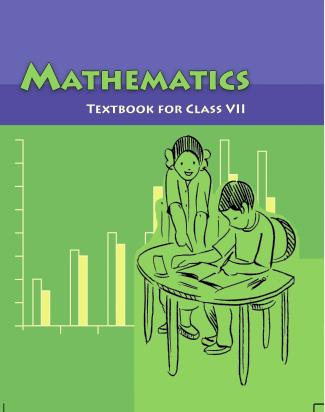## NCERT Solutions for Class 7 Maths Practical Geometry

###### Class –VII Mathematics (Ex. 10.6)

Below are given the measures of certain sides and angles of triangles. Identify those which cannot be constructed and, say why you cannot construct them. Construct rest of the triangle.

Triangle Given measurements

1. {tex}\Delta {/tex}ABC {tex}m\angle {/tex}A = {tex}85^\circ {\text{ ;}}{/tex} {tex}m\angle {/tex}B = {tex}115^\circ {\text{ ;}}{/tex} AB = 5 cm

2. {tex}\Delta {/tex}PQR {tex}m\angle {/tex}Q = {tex}30^\circ {\text{ ;}}{/tex} {tex}m\angle {/tex}R = {tex}60^\circ {\text{ ;}}{/tex} QR = 4.7 cm

3. {tex}\Delta {/tex}ABC {tex}m\angle {/tex}A = {tex}70^\circ {\text{ ;}}{/tex} {tex}m\angle {/tex}B = {tex}50^\circ {\text{ ;}}{/tex} AC = 3 cm

4. {tex}\Delta {/tex}LMN {tex}m\angle {/tex}L = {tex}60^\circ {\text{ ;}}{/tex} {tex}m\angle {/tex}N = {tex}120^\circ {\text{ ;}}{/tex} LM = 5 cm

5. {tex}\Delta {/tex}ABC BC = 2 cm; AB = 4 cm; AC = 2 cm

6. {tex}\Delta {/tex}PQR PQ = 3.5 cm; QR = 4 cm; PR = 3.5 cm

7. {tex}\Delta {/tex}XYZ XY = 3 cm; YZ = 4 cm; XZ = 5 cm

8. {tex}\Delta {/tex}DEF DE = 4.5 cm; EF = 5.5 cm; DF = 4 cm

###### Miscellaneous Questions

1.In {tex}\Delta {/tex}ABC, {tex}m\angle {/tex}A = {tex}85^\circ ,m\angle {/tex}B = {tex}115^\circ ,{/tex} AB = 5 cm Construction of {tex}\Delta {/tex}ABC is not possible because {tex}m\angle {/tex}A = {tex}85^\circ + m\angle {/tex}B = {tex}200^\circ ,{/tex} and we know that the sum of angles of a triangle should be {tex}180^\circ .{/tex}

###### 2.To construct: {tex}\Delta {/tex}PQR where {tex}m\angle {/tex}Q = {tex}{30^ \circ },{/tex}{tex}m\angle {/tex}R = {tex}{60^ \circ }{/tex} and QR = 4.7 cm.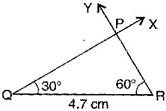Steps of construction:

1. Draw a line segment QR = 4.7 cm.

(a) At point Q, draw {tex}\angle {/tex}XQR = {tex}{30^ \circ }{/tex} with the help of compass.

(b) At point R, draw {tex}\angle {/tex}YRQ = {tex}{60^ \circ }{/tex} with the help of compass.

(c) QX and RY intersect at point P.

It is the required triangle PQR.

3. We know that the sum of angles of a triangle is {tex}180^\circ .{/tex}

{tex}\therefore {/tex} {tex}m\angle {/tex}A + {tex}m\angle {/tex}B + {tex}m\angle {/tex}C = {tex}180^\circ {/tex}

{tex} \Rightarrow {/tex} {tex}70^\circ + 50^\circ + m\angle {/tex}C = {tex}180^\circ {/tex}

{tex} \Rightarrow {/tex} {tex}120^\circ {/tex} + {tex}m\angle {/tex}C = {tex}180^\circ {/tex}

{tex} \Rightarrow {/tex} {tex}m\angle {/tex}C = {tex}180^\circ {/tex} – {tex}120^\circ {/tex}

{tex} \Rightarrow {/tex} {tex}m\angle {/tex}C = {tex}60^\circ {/tex}

To construct: {tex}\Delta {/tex}ABC where {tex}m\angle {/tex}A = {tex}70^\circ {/tex}, {tex}m\angle {/tex}C = {tex}60^\circ {/tex} and AC = 3 cm.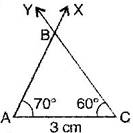Steps of construction:

(a) Draw a line segment AC = 3 cm.

(b) At point C, draw {tex}\angle {/tex}YCA = {tex}{60^ \circ }.{/tex}

(c) At point A, draw {tex}\angle {/tex}XAC = {tex}70^\circ .{/tex}

(d) Rays XA and YC intersect at point B

It is the required triangle ABC.

4. In {tex}\Delta {/tex}LMN , {tex}m\angle {/tex}L = {tex}{60^ \circ },{/tex}{tex}m\angle {/tex}N = {tex}120^\circ ,{/tex} LM = 5 cm

This {tex}\Delta {/tex}LMN is not possible to construct because {tex}m\angle {/tex}L + {tex}m\angle {/tex}N = {tex}60^\circ + 120^\circ = 180^\circ {/tex} which forms a linear pair.

5. {tex}\Delta {/tex}ABC, BC = 2 cm, AB = 4 cm and AC = 2 cm

This {tex}\Delta {/tex}ABC is not possible to construct because the condition is

Sum of lengths of two sides of a triangle should be greater than the third side.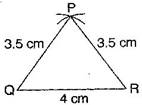AB < BC + AC {tex} \Rightarrow {/tex} 4 < 2 + 2 {tex} \Rightarrow {/tex} 4 = 4,

NCERT Solutions for Class 7 Maths Exercise 10.6

###### 6. To construct: {tex}\Delta {/tex}PQR where PQ = 3.5 cm, QR = 4 cm and PR = 3.5 cm

Steps of construction:

(a) Draw a line segment QR = 4 cm.

(b) Taking Q as centre and radius 3.5 cm, draw an arc.

(c) Similarly, taking R as centre and radius 3.5 cm, draw an another arc which intersects the first arc at point P.

It is the required triangle PQR.

NCERT Solutions for Class 7 Maths Exercise 10.6

###### 7. To construct: A triangle whose sides are XY = 3 cm, YZ = 4 cm and XZ = 5 cm.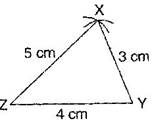Steps of construction:

(a) Draw a line segment ZY = 4 cm.

(b) Taking Z as centre and radius 5 cm, draw an arc.

(c) Taking Y as centre and radius 3 cm, draw another arc.

(d) Both arcs intersect at point X.

It is the required triangle XYZ.

NCERT Solutions for Class 7 Maths Exercise 10.6

###### 8.To construct: A triangle DEF whose sides are DE = 4.5 cm, EF = 5.5 cm and DF = 4 cm.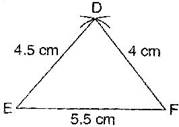Steps of construction:

(a) Draw a line segment EF = 5.5 cm.

(b) Taking E as centre and radius 4.5 cm, draw an arc.

(a) Taking F as centre and radius 4 cm, draw an another arc which intersects the first arc at point D.

It is the required triangle DEF.

## NCERT Solutions for Class 7 Maths Exercise 10.6

NCERT Solutions Class 7 Maths PDF (Download) Free from myCBSEguide app and myCBSEguide website. Ncert solution class 7 Maths includes text book solutions from Class 7 Maths Book . NCERT Solutions for CBSE Class 7 Maths have total 15 chapters. 7 Maths NCERT Solutions in PDF for free Download on our website. Ncert Maths class 7 solutions PDF and Maths ncert class 7 PDF solutions with latest modifications and as per the latest CBSE syllabus are only available in myCBSEguide.

## CBSE app for Students

To download NCERT Solutions for Class 7 Maths, Social Science Computer Science, Home Science, Hindi English, Maths Science do check myCBSEguide app or website. myCBSEguide provides sample papers with solution, test papers for chapter-wise practice, NCERT solutions, NCERT Exemplar solutions, quick revision notes for ready reference, CBSE guess papers and CBSE important question papers. Sample Paper all are made available through the best app for CBSE students and myCBSEguide website.### Test Generator

Create question paper PDF and online tests with your own name & logo in minutes.### myCBSEguide

Question Bank, Mock Tests, Exam Papers, NCERT Solutions, Sample Papers, Notes